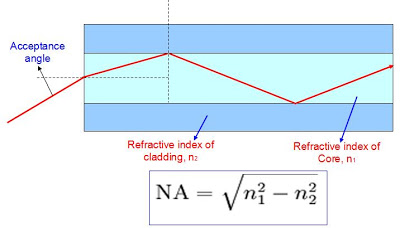# Numerical aperture in fiber optics

Numerical Aperture is defined as the maximum acceptance angle to allow and transmit light by an optical fiber. Multimode fibers allow propagation of more than 100 modes through them. The factors deciding the number modes that can be travelled though a multimode fiber are the core diameter and the numerical aperture.

As the core size and Numerical aperture (NA) increase, the number of modes increases. Typical values of fiber core size ranges from 50 to 100 micrometers and Numerical aperture value ranges from 0.20 to 0.29. For a 50/125 micrometer multimode fiber the numerical value specified generally is 0.20 with a tolerance of +/- 0.015. For a multimode fiber having core diameter of 62.5/125 micrometers, the numerical aperture value as a standard practice is 0.275 nominal with a tolerance of +/- 0.015.

Light beam enters into a multimode optical fiber at an angle. The characteristic of multimode fiber is that it allows propagation of light that enters within a certain cone. This cone is known in fiber optic technology as the acceptance cone of optical fiber. ½ of the angle of this cone is called the acceptance angle. The acceptance angle is determined only by the indices of refraction for a multimode optical fiber having step-index profile.where n1 is the refractive index of the fiber core, and n2 is the refractive index of cladding.

The relation between the acceptance angle and the numerical aperture becomes an approximation when the above formula is used. It is common that some optical fiber manufacturers refer Numerical aperture using the above formula for single mode optical fiber.

For a single mode fiber, numerical aperture is not a particularly required value, but some specifications ask suppliers to quote the numerical aperture value for single mode optical fiber also. Acceptance angle in a single mode optical fiber is different from that of a multimode fiber and hence numerical aperture of a single mode fiber can not be determined only by using refractive indices of cladding and core alone. The number of bound modes, the mode volume, is related to the normalized frequency and thus to the NA.

Having a large core size and a higher Numerical Aperture have several advantages. Light beam can be launched into a multimode fiber more easily. Larger Numerical Aperture and core make easy splicing.

QuestTel shall have no liability for any error or damage of any kind resulting from the use of this document.